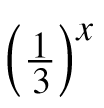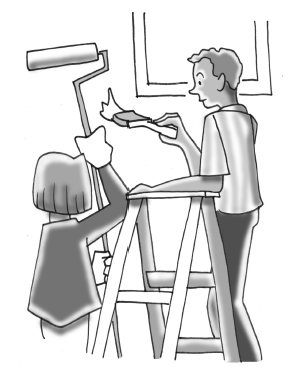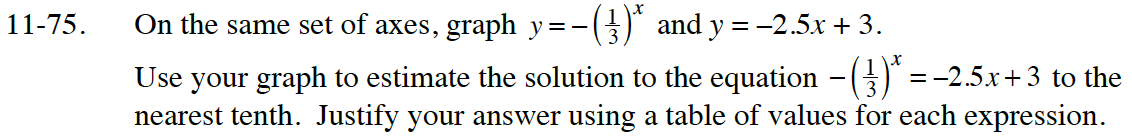Home > CC4 > Chapter 11 > Lesson 11.2.3 > Problem11-75

11-75.

On the same set of axes, graph y = −and y = −2.5x + 3. Use your graph to estimate the solution to the equation −= −2.5x + 3 to the nearest tenth. Justify your answer using a table of values for each expression. 11-75 HW eTool (Desmos). Homework Help ✎Use the eTool below to graph the equations.
Click the link at the right to view full version of the eTool: Int1 11-75 HW eTool.